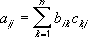## Excel Office

Excel How Tos, Tutorials, Tips & Tricks, Shortcuts

# MMULT function: Description, Usage, Syntax, Examples and Explanation

## What is MMULT function in Excel?

MMULT function is one of the Math and Trig functions in Microsoft Excel that returns the matrix product of two arrays. The result is an array with the same number of rows as array1 and the same number of columns as array2.

Worked Example:   Count rows that contain specific values in Excel

## Syntax of MMULT function

MMULT(array1, array2)

The MMULT function syntax has the following arguments:

• Array1, Array2: The arrays you want to multiply.

## MMULT formula explanation

• The number of columns in Array1 must be the same as the number of rows in Array2, and both arrays must contain only numbers.
• Array1 and Array2 can be given as cell ranges, array constants, or references.
• MMULT returns the #VALUE! error when:
• Any cells are empty or contain text.
• The number of columns in Array1 is different from the number of rows in Array2.
• The matrix product array a of two arrays b and c is:where i is the row number, and j is the column number.
• Formulas that return arrays must be entered as array formulas.
Worked Example:   Count rows with at least n matching values